# SAS/STAT Examples

## Fitting Bayesian Zero-Inflated Poisson Regression Models with the MCMC Procedure

Contents | SAS Program | PDF

## Overview

This example illustrates fitting Bayesian zero-inflated Poisson (ZIP) models to zero-inflated count data with the experimental MCMC procedure. ZIP models are often used when count data show an excess number of zeros, which in turn causes overdispersion. Consider survey data collected at a state park concerning the number of fish that visitors had caught in the last six months. People from two different populations provided information: those who attended the park and fished, and those who attended the park and did not fish. Zero fish caught has a different meaning for the two populations.

## Analysis

Count data frequently display overdispersion and excess zeros, which motivates zero-inflated count models (Lambert; 1992 ; Greene; 1994 ) . Zero-inflated count models offer a way of modeling the excess zeros in addition to allowing for overdispersion in a standard parametric model. Zero inflation arises when one mechanism generates only zeros and the other process generates both zero and nonzero counts.

Zero-inflated models can be expressed as a two-component mixture model where one component has a degenerate distribution at zero and the other is a count model. More formally, a zero-inflated model can be written as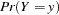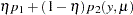where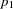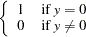( 1 )

and where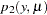is a standard count model with mean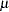, support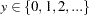, and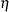is a mixture proportion with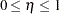.

The following hypothetical data represents the number of fish caught by visitors at a state park. Variables are created for the visitor’s age and gender. Two dummy variables, FEMALE and MALE, are created to indicate the gender.

```   data catch;
input gender \$ age count @@;
if gender = 'F' then do;
female = 1; male = 0;
end;
else do;
female = 0; male = 1;
end;
obs = _N_;
datalines;
F 54 18 M 37 0 F 48 12 M 27 0
M 55 0 M 32 0 F 49 12 F 45 11
M 39 0 F 34 1 F 50 0 M 52 4
M 33 0 M 32 0 F 23 1 F 17 0
F 44 5 M 44 0 F 26 0 F 30 0
F 38 0 F 38 0 F 52 18 M 23 1
F 23 0 M 32 0 F 33 3 M 26 0
F 46 8 M 45 5 M 51 10 F 48 5
F 31 2 F 25 1 M 22 0 M 41 0
M 19 0 M 23 0 M 31 1 M 17 0
F 21 0 F 44 7 M 28 0 M 47 3
M 23 0 F 29 3 F 24 0 M 34 1
F 19 0 F 35 2 M 39 0 M 43 6
;
```

Although these data appear to be a likely candidate for a ZIP model, you typically begin with a standard analysis and evaluate the evidence for overdispersion.

## Bayesian Poisson Regression Model

Suppose you want to fit a Bayesian Poisson regression model for the number of fish caught with density as follows: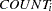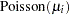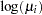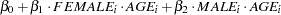( 2 )

for the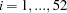surveyed park visitors.

The likelihood function for each of the counts and corresponding covariates is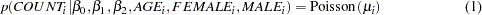( 3 )

where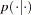denotes a conditional probability density. The Poisson density is evaluated at the specified value of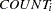and corresponding mean parameter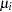. The three parameters in the likelihood are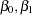, and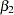, which correspond to an intercept, slope for age for females, and slope for age for males, respectively.

Suppose the following prior distributions are placed on the three parameters: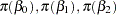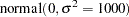( 4 )

where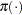indicates a prior distribution. The diffuseprior expresses your lack of knowledge about the regression parameters.

Using Bayes’ theorem, the likelihood function and prior distributions determine the posterior distribution of, andas follows: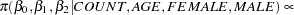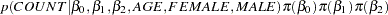PROC MCMC obtains samples from the desired posterior distribution, which is determined by the prior and likelihood specified. It does not require the form of the posterior distribution.

The goodness-of-fit Pearson chi-square statistic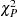as given in McCullagh and Nelder ( 1989 ) , is calculated to assess model fit: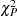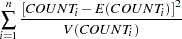( 5 )

for a Poisson likelihood where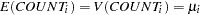withas defined in Equation 2 ,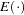is the expectation, and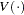is the variance. If there is no overdispersion, the Pearson statistic would roughly equal the number of observations in the data set minus the number of parameters in the model.

The following SAS statements use the diffuse prior distributions to fit the Bayesian Poisson regression model and calculate the fit statistic.

```   ods graphics on;
proc mcmc data=catch seed=1181 nmc=100000 thin=10
propcov=quanew monitor =(_parms_ Pearson);
ods select Parameters PostSummaries PostIntervals tadpanel;
parms beta0 0 beta1 0 beta2 0;
prior beta: ~ normal(0,var=1000);
mu = exp(beta0 + beta1*female*age + beta2*male*age);
model count ~ poisson(mu);
if obs = 1 then Pearson = 0;
Pearson =  Pearson + ((count - mu)**2/mu);
run;
ods graphics off;
```

The PROC MCMC statement invokes the procedure and specifies the input data set. The SEED= option specifies a seed for the random number generator, which guarantees the reproducibility of the random stream. The NMC= option specifies the number of posterior simulation iterations. The THIN=10 option controls the thinning of the Markov chain and specifies that one of every 10 samples is kept. The PROPCOV=QUANEW option initializes the Markov chain at the posterior mode and uses the estimated inverse Hessian matrix as the initial proposal covariance matrix in the random walk Metropolis algorithm. The MONITOR= option specifies a list of symbols (which can be either parameters or functions of the parameters in the model) for which inference is to be done. The symbol _parms_ is a shorthand for all model parameters—in this case,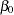,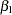, and. The symbol Pearson refers to the Pearson chi-square statistic, which is a function of the data and parameters.

The PARMS statement puts all three parameters,, andin a single block and assigns initial values to each of them. The PRIOR statement specifies priors for all the parameters. The notation beta: in the PRIOR statement is a shorthand for all variables that start with ' beta '. In this example, it includes beta0 , beta1 , and beta2 . The shorthand notation is not necessary, but it keeps your code succinct. The assignment statement for mu calculatesin the Poisson model, as given in Equation 2 . The MODEL statement specifies the likelihood function for COUNT, as given in Equation 3 .

The next two lines of statements use the posterior samples of mu and the data set variable count to calculate the Pearson chi-square statistic. The IF statement resets the value of Pearson to be zero at the top of the data set (that is, when the data set variable obs is 1). As PROC MCMC cycles through the data set at each iteration, the procedure cumulatively adds the Pearson chi-square statistic over each value of count . By the end of the data set, you obtain the Pearson chi-square statistic, as defined in Equation 5 .

It is essential to examine the convergence of the Markov chains before you proceed with posterior inference in Bayesian analysis. With ODS Graphics turned on, PROC MCMC produces graphs at the end of the procedure output which allow you to visually examine the convergence of the chain. See Figure 1 . Inferences should not be made if the Markov chain has not converged.

Figure 1 Bayesian Poisson Model Diagnostic Plots for, and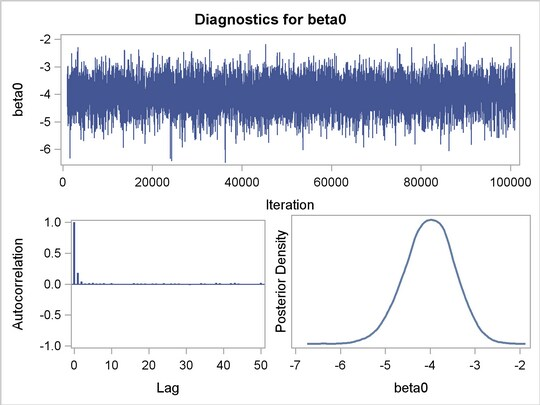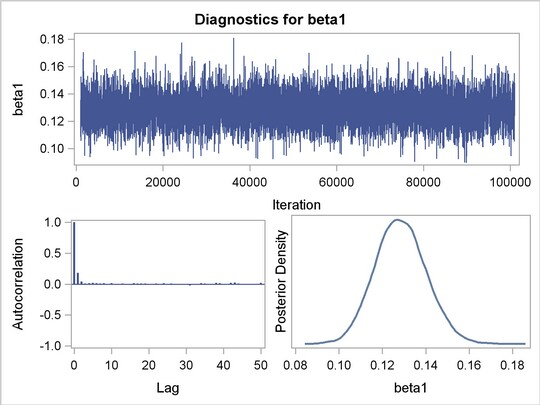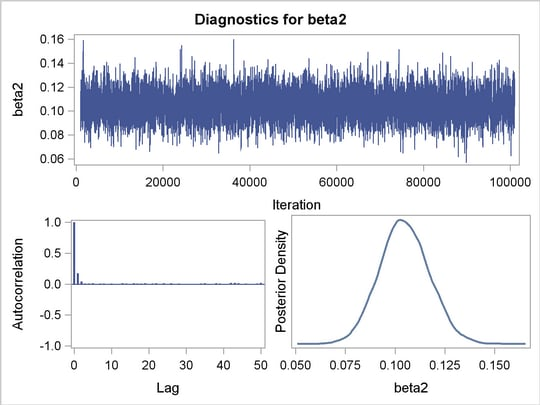Figure 1 displays convergence diagnostic plots for,, and. The trace plots show that the mean of the Markov chain is constant over the graph and is stabilized. The chain was able to traverse the support of the target distribution, and the mixing is good. The trace plots show that the Markov chain appears to have reached stationary distributions.

The autocorrelation plots indicate low autocorrelation and efficient sampling. The kernel density plots show smooth, unimodal posterior marginal distributions for each parameter.

PROC MCMC produces formal diagnostic tests by default, but they are omitted here because an informal check on the chains, autocorrelation, and posterior density plots show desired stabilization and convergence.

The "Parameters" tables, shown in Figure 2 , lists the names of the parameters, the sampling method used, the starting values, and the prior distributions.

Figure 2 Bayesian Model Information
The MCMC Procedure
Parameters
Parameter Sampling
Method
Initial
Value
Prior Distribution
beta0 N-Metropolis 0 normal(0,var=1000)
beta1 N-Metropolis 0 normal(0,var=1000)
beta2 N-Metropolis 0 normal(0,var=1000)

Figure 3 displays summary and interval statistics for each parameter’s posterior distribution. PROC MCMC also calculates the sampled value of the Pearson chi-square at each iteration and produces posterior summary statistics for it.

Figure 3 Posterior Model Summary of Poisson Regression
The MCMC Procedure

Posterior Summaries
Parameter N Mean Standard
Deviation
Percentiles
25% 50% 75%
beta0 10000 -4.0186 0.5551 -4.3787 -4.0024 -3.6411
beta1 10000 0.1284 0.0117 0.1204 0.1281 0.1361
beta2 10000 0.1047 0.0125 0.0963 0.1043 0.1129
Pearson 10000 92.2674 12.2613 83.3871 90.0545 98.8112

Posterior Intervals
Parameter Alpha Equal-Tail Interval HPD Interval
beta0 0.050 -5.1475 -2.9799 -5.0903 -2.9400
beta1 0.050 0.1064 0.1518 0.1065 0.1518
beta2 0.050 0.0808 0.1295 0.0799 0.1283
Pearson 0.050 74.8858 121.7 72.8727 116.1

With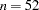and three model parameters, the sampled value 92.2674 of the Pearson chi-square statistic is much greater than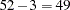, providing evidence of overdispersion.

Zero inflation is a likely cause of this overdispersion. A Bayesian ZIP model accounts for the extra zeros and potentially provides a better fit to the data.

### Bayesian ZIP Regression Model

You can write a Bayesian ZIP regression model for the number of fish caught as follows: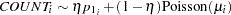where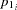is defined in Equation 1 ,is defined in Equation 2 , andfor thesurveyed visitors. The model is a weighted average of the degenerate function (which places all mass at zero) and the Poisson regression.

The likelihood function for each of the counts and corresponding covariates is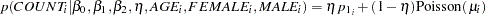( 6 )

wheredenotes a conditional probability density and the Poisson density is evaluated at the specified value ofand corresponding mean parameter. The degenerate distributionis one when COUNT equals zero and remains zero for a COUNT value greater than zero. The four parameters in the likelihood are,, and, which correspond to an intercept, slope for age for females, slope for age for males, and the mixture proportion, respectively.

Suppose again that the three regression parameters have the same diffuse, normal priors as in Equation 4 . The mixture proportion has a uniform(0,1) prior distribution.

The Pearson chi-square statistic for the ZIP model is calculated as in Equation 5 , but now the mean and the variance respectively are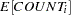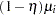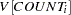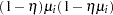The following SAS statements use the prior distributions to fit the Bayesian ZIP regression model and calculate the Pearson chi-square statistic.

```   ods graphics on;
proc mcmc data=catch seed =1181 nmc=100000 thin=10
propcov=quanew monitor =(_parms_ Pearson);
ods select Parameters PostSummaries PostIntervals tadpanel;
parms beta0 0 beta1 0 beta2 0 eta .3;
prior beta: ~ normal(0,var=1000);
prior eta ~ uniform(0,1);
mu=exp(beta0 + beta1*female*age + beta2*male*age);
llike=log(eta*(count eq 0) + (1-eta)*pdf("poisson",count,mu));
model general(llike);
if obs = 1 then Pearson = 0;
mean = (1 - eta)*mu;
sigma2 = (1 - eta)*mu*(1 + eta*mu);
Pearson =  Pearson + ((count - mean)**2/sigma2);
run;
ods graphics off;
```

The parameterand its starting value are added to the PARMS statement with the regression parameters and their starting values. The PRIOR statement remains the same for the regression parameters, but an additional PRIOR statement is needed for the mixture proportion.

The assignment statement for mu calculates the expected value of COUNT in the Poisson model, as given in Equation 2 . The assignment statement for llike evaluates the log density of Equation 6 . The expression (count eq 0) in llike acts as an indicator variable for the degenerate distribution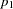; it is one when COUNT equals zero and zero for values of COUNT greater than zero. The MODEL statement specifies that llike is the log likelihood for each observation in the model.

The Pearson chi-square statistic is calculated according to Equation 5 ; the moments are evaluated in the mean and sigma2 assignment variables.

Figure 4 Bayesian ZIP Diagnostic Plots for,, and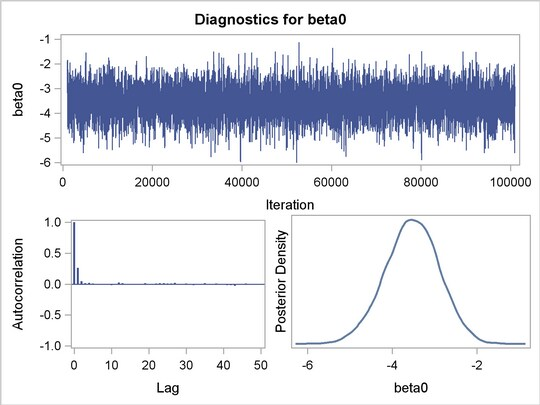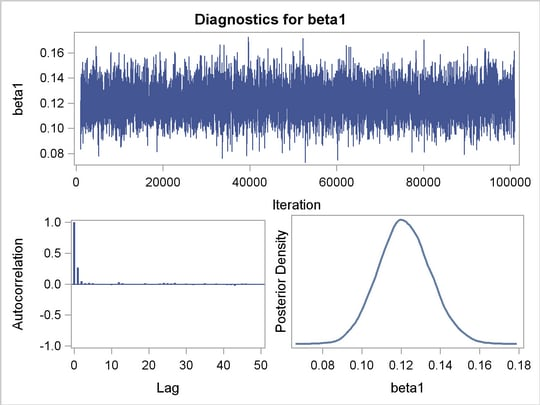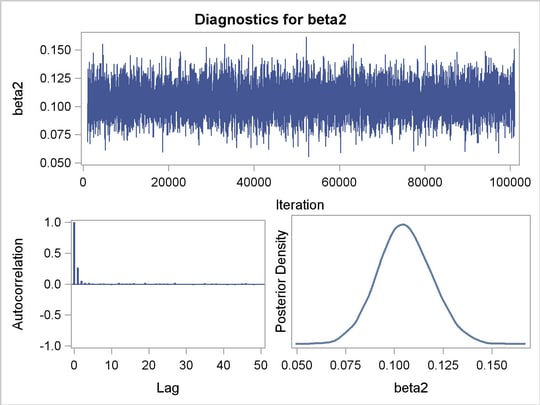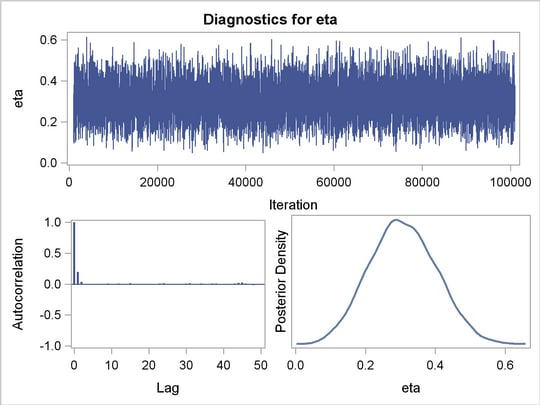The diagnostic plots for the regression parameters and mixture proportion are illustrated in Figure 4 . They show the desired convergence, low autocorrelation, and smooth unimodal marginal posterior densities for the parameters.

Figure 5 displays the "Parameters" table for the ZIP regression model. The "Parameters" table now includes information about the mixture proportion parameter.

Figure 5 Bayesian ZIP Regression Model Information
The MCMC Procedure

Parameters
Parameter Sampling
Method
Initial
Value
Prior Distribution
beta0 N-Metropolis 0 normal(0,var=1000)
beta1 N-Metropolis 0 normal(0,var=1000)
beta2 N-Metropolis 0 normal(0,var=1000)
eta N-Metropolis 0.3000 uniform(0,1)

Figure 6 displays summary and interval statistics for each parameter’s posterior distribution. PROC MCMC also calculates the sampled value of the Pearson chi-square at each iteration. This statistic in the Bayesian ZIP model is greatly reduced with a value of 48.8650, which suggest a much better fit compared to its value of 92.2674 in the Poisson model.

Figure 6 Posterior Summary of Bayesian ZIP Regression
The MCMC Procedure

Posterior Summaries
Parameter N Mean Standard
Deviation
Percentiles
25% 50% 75%
beta0 10000 -3.5345 0.6349 -3.9516 -3.5278 -3.0980
beta1 10000 0.1217 0.0132 0.1127 0.1214 0.1304
beta2 10000 0.1054 0.0138 0.0961 0.1051 0.1146
eta 10000 0.3074 0.0936 0.2420 0.3050 0.3719
Pearson 10000 48.8650 10.2696 41.4549 47.2985 54.4092

Posterior Intervals
Parameter Alpha Equal-Tail Interval HPD Interval
beta0 0.050 -4.8193 -2.3170 -4.7908 -2.2925
beta1 0.050 0.0963 0.1485 0.0955 0.1476
beta2 0.050 0.0786 0.1331 0.0785 0.1328
eta 0.050 0.1296 0.4926 0.1290 0.4915
Pearson 0.050 33.7757 73.5911 31.6653 68.8728

Modeling the catch data set with a Bayesian ZIP regression model accounts for the zero inflation and removes the overdispersion in the Poisson regression model. Figure 6 shows the posterior parameter summaries in addition to the lowered Pearson chi-square statistic. The posterior mean of the mixture probability is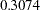for the zero-inflated component and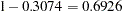for the Poisson regression component. The posterior parameter means ofandare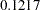and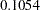, respectively. That is, an increase in one year of age is estimated to be associated with a change in the mean number of fish caught by females and males by a factor of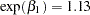and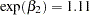, respectively. For this model, choice of priors, and data set, females catch more fish with age than males.

## References

Greene, W. H. (1994), Accounting for Excess Zeros and Sample Selection in Poisson and Negative Binomial Regression Models , working paper 94-10, New York University, Leonard N. Stern School of Business, Department of Economics, available at http://ideas.repec.org/p/ste/nystbu/94-10.html .

Lambert, D. (1992), “Zero-Inflated Poisson Regression Models with an Application to Defects in Manufacturing,” Technometrics , 34, 1–14.

McCullagh, P. and Nelder, J. A. (1989), Generalized Linear Models , Second Edition, London: Chapman & Hall.